# MySQL JOINS

## Understanding JOINs in MySQL

A join enables you to retrieve records from two (or more) logically related tables in a single result set.

JOIN clauses are used to return the rows of two or more queries using two or more tables that shares a meaningful relationship based on a common set of values.

These values are usually the same column name and datatype that appear in both the participating tables being joined. These columns, or possibly a single column from each table, are called the join key or common key.

Mostly but not all of the time, the join key is the primary key of one table and a foreign key in another table. The join can be performed as long as the data in the columns are matching.

It can be difficult when the join involving more than two tables. It is a good practice to think of the query as a series of two table joins when the involvement of three or more tables in joins.

MySQL JOIN Syntax:

MySQL supports the following JOIN syntaxes for the table_references (A table reference is also known as a join expression.) part of SELECT statements and multiple-table UPDATE and DELETE statements :

```table_references:
escaped_table_reference [, escaped_table_reference] ...

escaped_table_reference:
table_reference
| { OJ table_reference }

table_reference:
table_factor
| join_table

table_factor:
tbl_name [PARTITION (partition_names)]
[[AS] alias] [index_hint_list]
| table_subquery [AS] alias
| ( table_references )

join_table:
table_reference [INNER | CROSS] JOIN table_factor [join_condition]
| table_reference STRAIGHT_JOIN table_factor
| table_reference STRAIGHT_JOIN table_factor ON conditional_expr
| table_reference {LEFT|RIGHT} [OUTER] JOIN table_reference join_condition
| table_reference NATURAL [{LEFT|RIGHT} [OUTER]] JOIN table_factor
join_condition:
ON conditional_expr
| USING (column_list)

index_hint_list:
index_hint [, index_hint] ...

index_hint:
USE {INDEX|KEY}
[FOR {JOIN|ORDER BY|GROUP BY}] ([index_list])
| IGNORE {INDEX|KEY}
[FOR {JOIN|ORDER BY|GROUP BY}] (index_list)
| FORCE {INDEX|KEY}
[FOR {JOIN|ORDER BY|GROUP BY}] (index_list)
index_list:
index_name [, index_name] ...```

Types of MySQL Joins :

• INNER JOIN
• LEFT JOIN
• RIGHT JOIN
• STRAIGHT JOIN
• CROSS JOIN
• NATURAL JOIN

Here is the sample tables table_A and table_B, which we have used to explain the technologies behind the joins.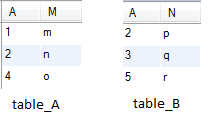MySQL INNER JOIN

The INNER JOIN is such a JOIN in which all rows can be selected from both participating tables as long as there is a match between the columns. Usage of INNER JOIN combines the tables. An INNER JOIN allows rows from either table to appear in the result if and only if both tables meet the conditions specified in the ON clause.

Example

Code:

``````SELECT * FROM table_A
INNER JOIN table_B
ON table_A.A=table_B.A;
```
```

Relational Algebra Expression: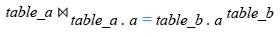Relational Algebra Tree: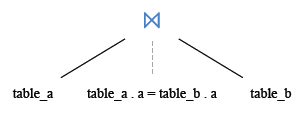MySQL LEFT JOIN

The LEFT JOIN is such a join which specifies that all records be fetched from the table on the left side of the join statement. If a record returned from the left table has no matching record in the table on the right side of the join, it is still returned, and the corresponding column from the right table returns a NULL value.

Example

Code:

``````SELECT * FROM table_A
LEFT JOIN table_B
ON table_A.A=table_B.A;
```
```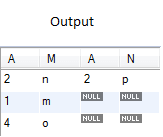MySQL RIGHT JOIN

The RIGHT JOIN is such a join which specifies that all records be fetched from the table on the right side of the join statement, even if the table on the left has no matching record. In this case, the columns from the left table return NULL values.

Example

Code:

``````SELECT * FROM table_A
RIGHT JOIN table_B
ON table_A.A=table_B.A;
```
```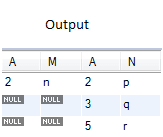MySQL STRAIGHT JOIN

An STRAIGHT_JOIN is such a join which scans and combines matching rows ( if specified any condition) which are stored in associated tables other wise it behaves like an INNER JOIN or JOIN of without any condition.

Example

Code:

``````SELECT * FROM table_A
STRAIGHT JOIN table_B;
```
```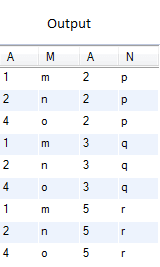MySQL CROSS JOIN

A CROSS JOIN is such a join which specifies the complete cross product of two tables. For each record in the first table, all the records in the second table are joined, creating a potentially huge result set. This command has the same effect as leaving off the join condition, and its result set is also known as a Cartesian product.

Example

Code:

``````SELECT * FROM table_A
CROSS JOIN table_B;
```
```MySQL NATURAL JOIN

A NATURAL JOIN is such a join that performs the same task as an INNER or LEFT JOIN, in which the ON or USING clause refers to all columns that the tables to be joined have in common.

Example

Code:

``````SELECT * FROM table_A
NATURAL JOIN table_B;
```
```

Relational Algebra Expression: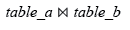Relational Algebra Tree: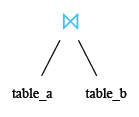Key points to remember

Click on the following to get the slides presentation -JOINS: SQL and Other Relational Databases

﻿